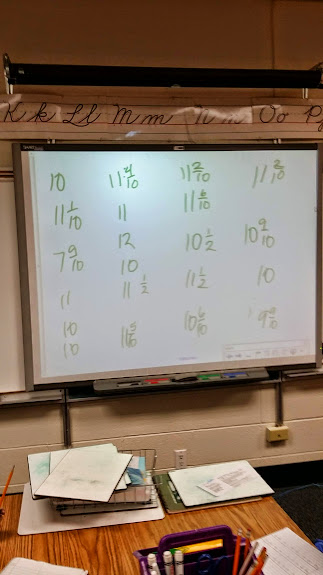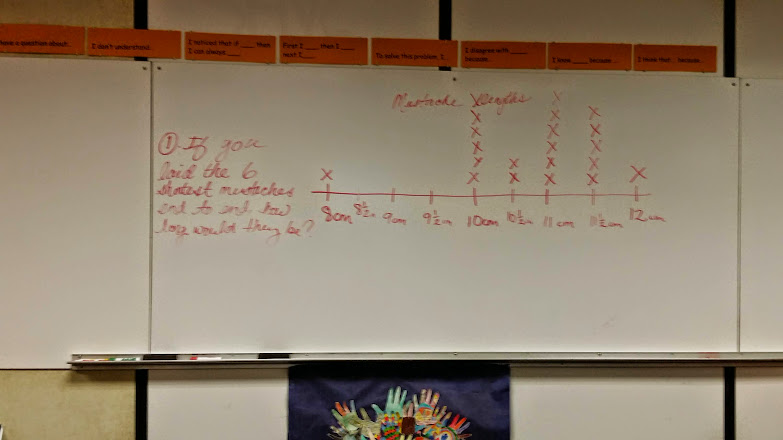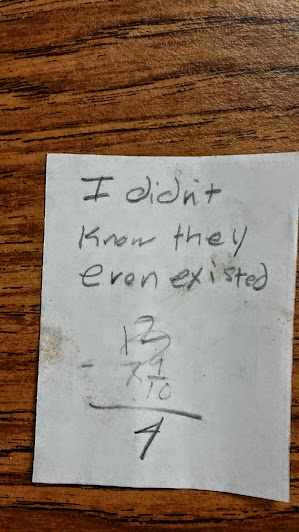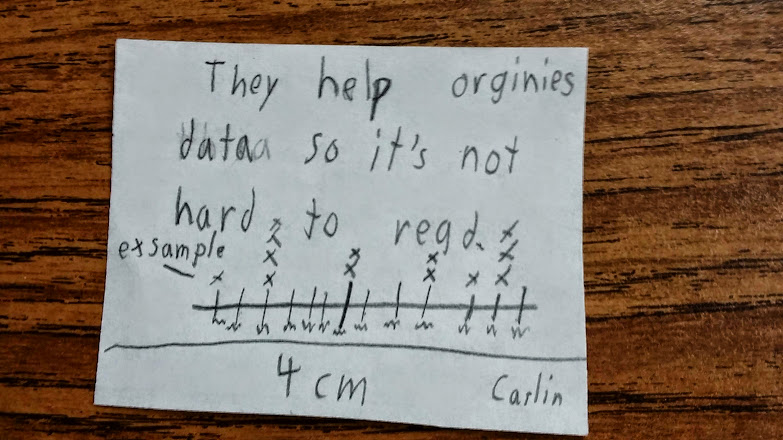# Who needs a Shave? - Line Plots

7 teachers like this lesson
Print Lesson

## Objective

SWBAT create line plots and display a data set of measurements in fractions of a unit.

#### Big Idea

In this lesson, students create line plots through a direct instruction approach and then answer questions using these line plots.

## Warm Up

5 minutes

For this warm up, I wanted students to have an opportunity to practice converting between millimeters, centimeters, meters, and kilometers.  I begin by showing students the power point.  I do not show this as a timed PowerPoint, rather display one slide and let students work on that particular problem.  Students answer each question on a whiteboard.  As students work, I circulate around the classroom observing and assisting as necessary.  When it looks like most students have answered, I ask student volunteers to share their answers and how or why they know they're right.

As students share, I re-state their responses emphasizing the operations used to convert.  I ask students how they know whether or not they would multiply or divide when converting within a system.  Some students are able to tell that when you go from a smaller unit to a larger unit, division works.  For example, 120 cm converted to meters, (smaller unit to larger unit) would work by using division to divide 120 by 100.  Other students have similar conceptual understanding but are not able to verbalize a general rule about converting to smaller from larger units, or from larger units to smaller units.

## Concept Development

45 minutes

In this lesson students work to make a line plot to display a data set of measurements in fractions of a unit or fractions of centimeters.  This lessons builds on students prior knowledge about fractions of numbers, as well as comparing fractions.  They will also solve problems involving addition and subtraction of fractions by using information presented in line plots.

I begin this lesson by telling students that often when we measure objects one way to tell more information about the objects is to graph the data gathered.  I tell students that today we will look at the data we gathered about our class mustaches from yesterday's lesson.

I show students the information gathered. (See photo below)  I ask students to tell me how much time they would need to solve a problem like this:

If we laid the six longest mustaches end to end, how many centimeters would they total?Students quickly start groaning and shouting out things like, "a long time!"  I ask a student volunteer to tell why that questions warrants so much time. Students realize that this type of question would take a long time simply because of the way the information is organized.

Next, I introduce the term line plot and tell students that one way to look at information is by graphing the frequency of data.  I tell students that line plots are one way to graph and organize data frequency.  I lead a short discussion about the term frequency and what that means for today's assignment. Students know that the word frequent means how many times something occurs, and are able to make the connections with the word frequency.

Then I tell students that we will create a line plot together in order to graph the frequency of lengths of our mustaches we created in the previous lesson. It is important that I create this line plot with my students because based on informal assessments, most of my students do not know what line plots are.  It is important that I re-teach my students about when line plots would be used. It is also important that I do this line plot with my students since this is the first time they are creating line plots with fractions as the values.

This is direct instruction approach. I chose to use direct instruction for this part of the lesson.  I ask students to look at the data on the board to find which mustache is the smallest.

On the other large whiteboard in my room, I draw a long line and place 1/2 centimeter marks underneath the line.  I tell students that the first number will be the smallest mustache and the we will mark 1/2 cm marks until we get to the length of the longest mustache.

Students do the same in their math notebooks. Then, as a class we decide where to place each "X" on the line plot. This is an important part of this lesson since the line plot has a scale of half centimeter increments.  As a class we look at our data and determine where each centimeter fraction would be placed. This is also a quick way for me to gauge if students are understanding where numbers are closest to. For example, I am looking to see that students know that 9  4/10 is closest to 9 1/2 centimeters and placed accordingly on the line plot. You can see in the photo below an example of the finished line plot.Next, I ask students if they could now solve the question about the six longest mustaches being laid end to end more quickly. Students respond with a unanimous YES!  I then write the question on the board and ask students to solve it.  I also ask students to find out the total length of the six shortest mustaches.

You can listen in as this student attempts to use his line plot to solve the question.  Notice how he is somewhat confused because of the number of mustaches that are 10 centimeters. He is confused about what to do with the shortest mustache because there happen to be 6 that are exactly 10 centimeters. At the end of the video, you can hear me tell the student I will be back to check on him.  He is experiencing some productive struggle. I help guide this student's thinking through questioning, but do not tell him what to do. I want him to struggle with the concept and question in order to own the knowledge for himself.

After students answer the initial question about the six longest mustaches, I lead a brief discussion about line lots. Some important questions I ask include:

How does the line plot help organize the data?

How would this help organize your thinking?

How many more are needed for your lowest measurement to equal your greatestmeasurement?

How many items total did you measure?

## Check for Understanding

5 minutes

To end this lesson, I ask students to complete an exit ticket and write something they learned today about line plots. You can see from the photo below, that this particular students responds by saying he/she didn't even know they existed.  This was common among many of my students.  Knowing this was a huge reason why I chose to do this lesson withe a direct instruction approach.You can also see another student's response. This student really connected with the idea that a line plot can be a tool to help organize information in order to analyze and evaluate information.